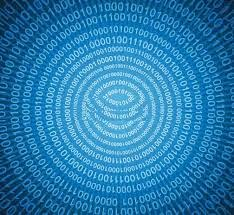首頁部落格Facebook論壇最新消息社群授權名單快速入口交換連結

## 2015年5月19日 星期二

### GB、TB、PB、EBGB、TB、PB、EB1Byte = 8 Bits
1 Kilobyte (KB)     = 1024 Bytes = 2^10 B ~ 10^3     （千）
1 Megabyte (MB)   = 1024 KB      = 2^20 B ~ 10^6     （百萬）
1 Gigabyte (GB)     = 1024 MB     = 2^30 B ~ 10^9     （十億）
1 Terabyte (TB)      = 1024 GB      = 2^40 B ~ 10^12   （兆）
1 Petabyte (PB)      = 1024 TB      = 2^50 B ~ 10^15   （千兆）
1 Exabyte (EB)        = 1024 PB      = 2^60 B ~ 10^18    （百京）
1 Zettabyte (ZB)     = 1024 EB      = 2^70 B ~ 10^21    （十垓ㄍㄞ）
1 Yottabyte (YB)   = 1024 ZB     = 2^80 B ~ 10^24   （秭ㄗˇ）

GB、TB、PB、EB
http://sbonny.blogspot.com/2015/05/gb-tb-pb-eb.html